# MOSFET structure and operation principles

### N-channel MOSFET

•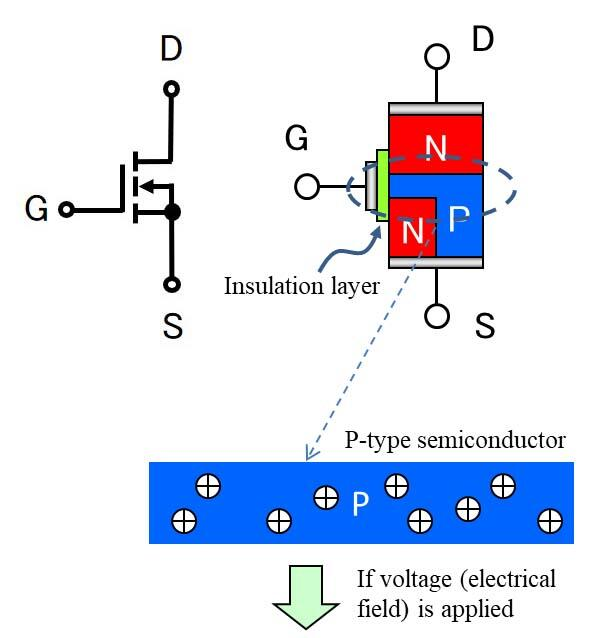The route between D→S is N→P→N and the N→P junction is backwards, so current cannot flow.
There are + electrical charges called “holes” distributed across P-type semiconductors.

•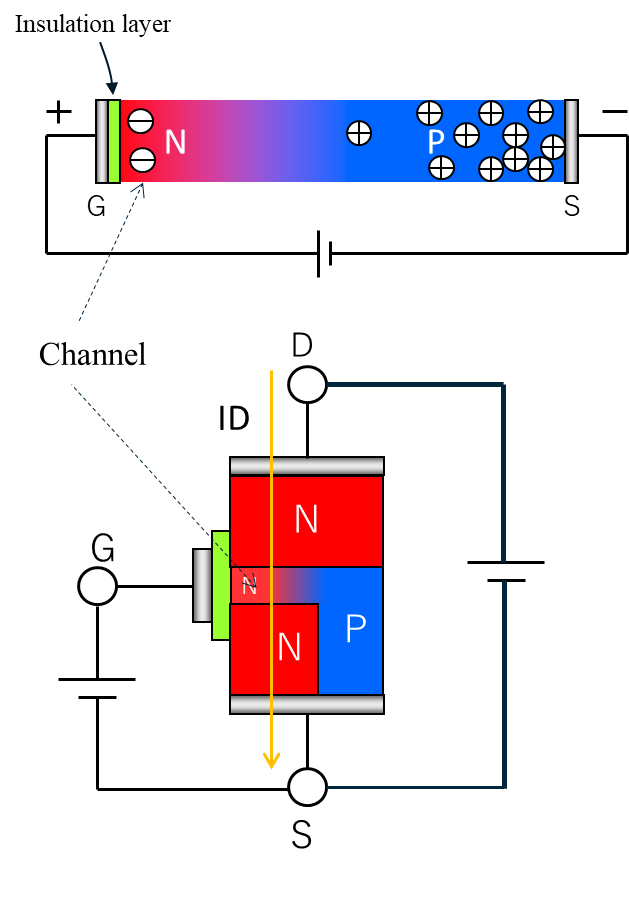If voltage is applied between G-S, the P layer directly below the gate will invert to N, creating an N-type semiconductor layer.This changes the N→P→N route to N→N→N, allowing current ID to flow.
This is a MOSFET in the “ON state”.

This N-type layer is called a “channel”, and because the channel is N-type, this is called an “N-channel MOSFET”.
The resistance of the channel is called the “channel resistance”.

### MOSFET gate threshold value (P8FE10SBK example)

Item

Rating

Unit

Explanation

Min.

Typ.

Max.

VTH

1.5

2.0

2.5

V

Voltage between G-S at which ID begins to flow (turns ON)

#### ① VGS=1V

•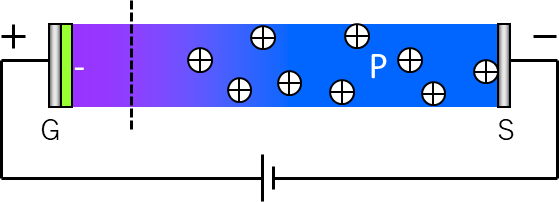Even if 1 V of voltage is applied between G-S, the channel will remain P and no current will flow.

#### ② VGS=2V

•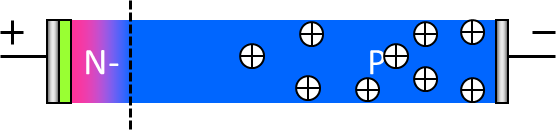If approximately 2 V of voltage is applied between G-S, then the channel will invert to N and current will begin to flow. ⇒This is the threshold value (VTH)

#### ③ VGS=4.5V

•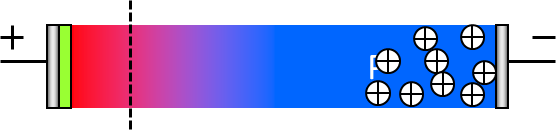In order to reliably lower the ON resistance, a voltage which is sufficiently larger than the VTH must be applied.
VGS = 85 mΩ at 4.5 V, VGS = 79 mΩ at 10 V

### Body diode

•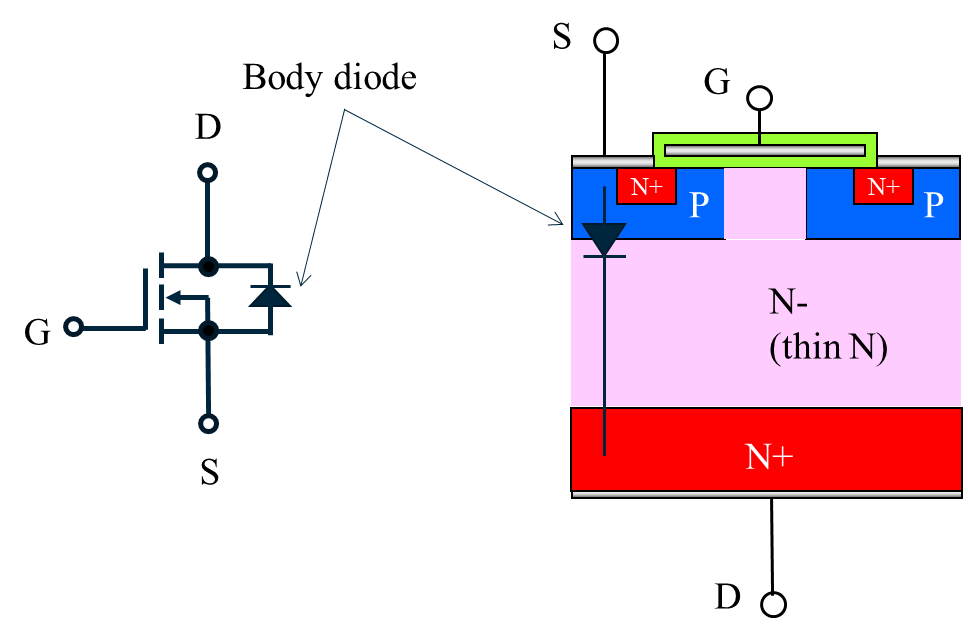As shown in the figure, the area between S⇒D is already a PN junction, or a diode, so current can flow at anytime.
This diode is called a “body diode”, and the MOSFET symbols are also written as shown in the figure.

### P-channel MOSFET

•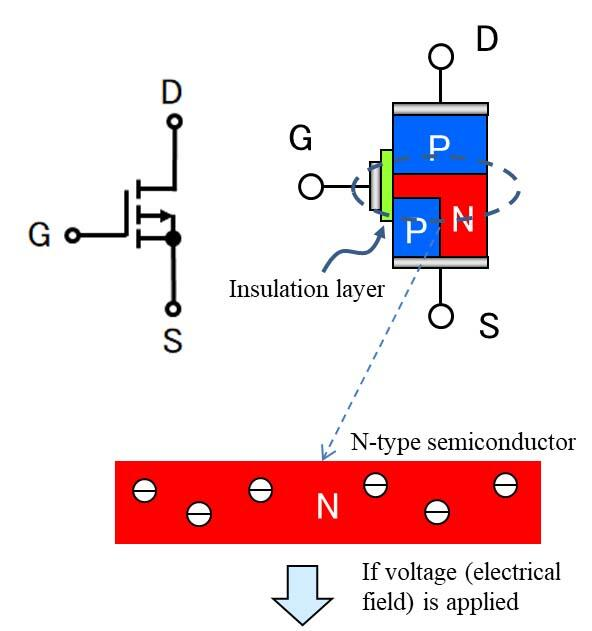The route between S→D is P→N→P and the N→P junction is backwards, so current cannot flow.

•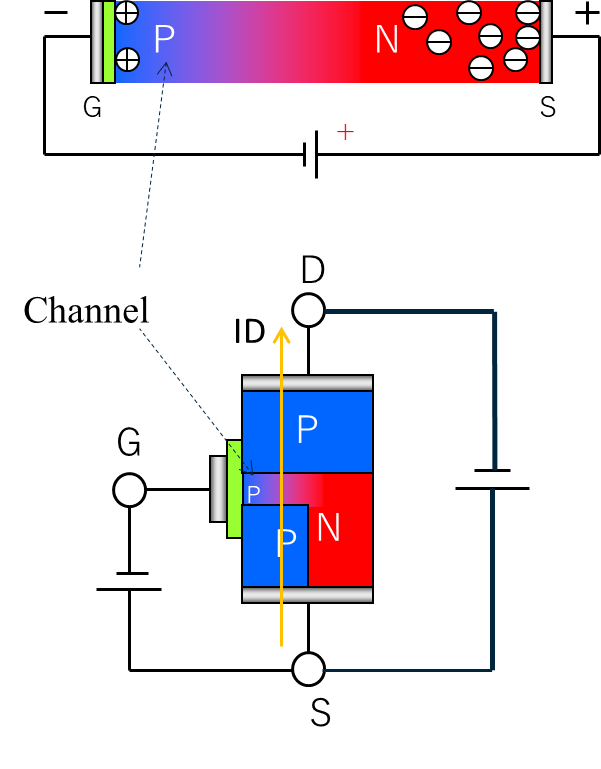If voltage is applied between G-S, the N layer directly below the gate will invert to P, creating an P-type semiconductor layer (channel).
This changes the P→N→P route to P→P→P, allowing current ID to flow.
This is a MOSFET in the “ON state”.
The channel is P-type, so this is called a P-channel MOSFET.

Because the current in P-type semiconductors is carried by “holes”, it is more difficult for current to flow in P-type semiconductors than in N-type semiconductors where current is carried by “electrons”.
In short, for the same size chip, the resistance will be higher than in an N-type.

### P-channel MOSFET characteristics

1. Negative voltage is applied between G-S to turn ON the MOSFET.
2. Has higher ON resistance than N-channel MOSFET.
3. The body diode is D→S orientation.

Due to these characteristics and from a usability standpoint, the majority of MOSFET used in the marketplace are N-channel MOSFET, and the product lineups of semiconductor manufacturers overwhelmingly feature N-channel MOSFET.

•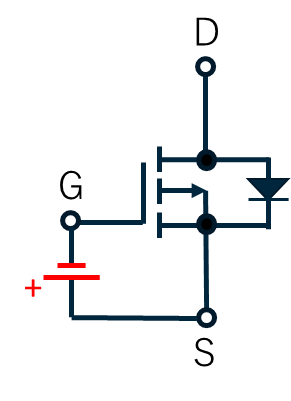P-Channel

#### P-channel MOSFET usage examples

•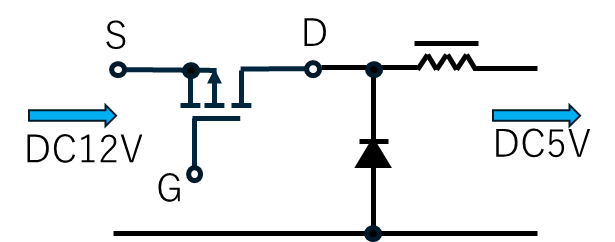Step-down DC/DC converters
•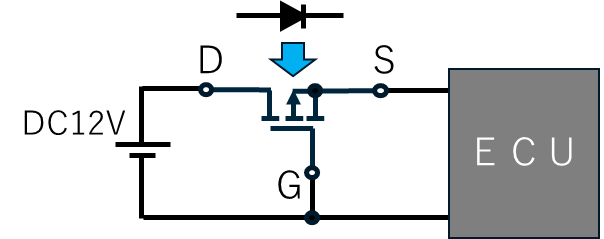Low loss conversion of reverse battery protection diodes